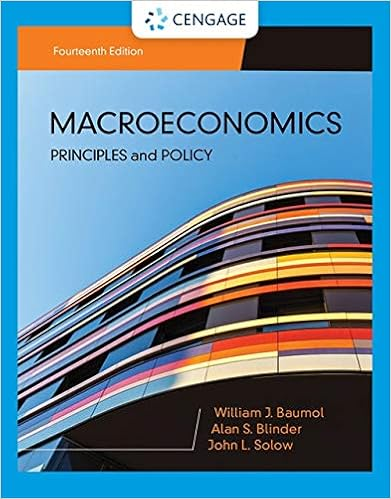# 2assume that a society consists of two types of

• Test Prep
• nbraswe
• 10
• 100% (1) 1 out of 1 people found this document helpful

This preview shows page 6 - 10 out of 10 pages.

##### We have textbook solutions for you!
The document you are viewing contains questions related to this textbook.The document you are viewing contains questions related to this textbook.
Chapter 9 / Exercise 4
Macroeconomics: Principles & Policy
Baumol/BlinderExpert Verified
2.Assume that a society consists of two types of workers. For type A, 3 million workers lose their jobs each year, and each one takes a year to find a new one. For type B, 36 million workers lose their jobs each year (3 million per month), and each takes one month to find a new job. Thus, at any given time, 6 million are unemployed in this economy. (10%, each 2.5 %) a. How many spells of unemployment occur each year in this economy? b. What percentage of the spells are only one month long? c. What is the average duration of each unemployment spell in this economy? d. Of all the months of unemployment, what fraction of total unemployment time is accounted for by the workers unemployed for a year? Page 6
##### We have textbook solutions for you!
The document you are viewing contains questions related to this textbook.The document you are viewing contains questions related to this textbook.
Chapter 9 / Exercise 4
Macroeconomics: Principles & Policy
Baumol/BlinderExpert Verified
3.Explain how each of the following events affects the monetary base, the money multiplier, and the money supply ( 20%, each part 4%) a. The Federal Reserve purchase long-term government bond during a quantitative easing. b. The Fed increases the interest rate it pays banks for holding reserves. c. Rumors about a bank run increase the amount of money people hold as currency rather than demand deposits. d. The Fed increases its lending to banks through its Term Auction Facility. e. The Fed increases the discount rate. Page 7
4.Assume that GDP ( Y ) is 5,000. Consumption ( C ) . is given by the equation C = 1,000 + 0.3( Y - T ). Investment ( I ) is given by the equation I = 2000 -50 r , where r is the real interest rate in percent. Taxes ( T ) are 1,000 and government spending ( G ) is 1,500. (20%, each part 5%) a. What are the equilibrium values of C , I , and b. What are the values of private saving, public saving, and national saving? c. Now assume that the government has a tax cut to reduce Taxes ( T ) to 500. What are the new equilibrium values of C , I , and d. Graphically illustrate how the above change in government policy would affect the loanable funds market. Be sure to label: i. the axes; ii. the curves; iii. the initial equilibrium values; iv. the direction curves shift; and v. the terminal equilibrium values. r ? r ? Page 8
Page 9
5.Consider two competitive economies that have the same quantities of labor ( L = 400) and capital ( K = 400), and the same technology ( A = 100). The economies of the countries are described by the following Cobb–Douglas production functions: North Economy: Y = A L .3 K .7 South Economy: Y = A L .7 K .3 a. Compute the output of the two economies. Which economy has the larger total production? Explain. (3%) b. Compute the marginal product of labor in each economy. In which economy is the marginal product of labor larger? Explain. (3%) c. In which economy is the real wage larger? Explain. (2%) d. In which economy is labor's share of income larger? Explain. (2%) Page 10
•••